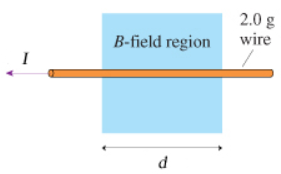# Problem: What magnetic field strength will levitate the 2.0 g wire in the figure (Figure 1) ? Assume that J = 1.5 A and d = 11 cm. What is the direction of the magnetic field?

###### FREE Expert Solution

Magnetic force:

$\overline{){{\mathbf{F}}}_{{\mathbf{B}}}{\mathbf{=}}{\mathbf{B}}{\mathbf{I}}{\mathbf{L}}{\mathbf{s}}{\mathbf{i}}{\mathbf{n}}{\mathbf{\theta }}}$

91% (279 ratings)###### Problem DetailsWhat magnetic field strength will levitate the 2.0 g wire in the figure (Figure 1) ? Assume that J = 1.5 A and d = 11 cm.

What is the direction of the magnetic field?